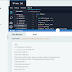> Gradle - Fabricating Systems Hacker-rank Hands-On Solutions - TECH UPDATE

# Gradle - Fabricating Systems Hacker rank Hands-On Solutions

The main agenda of these solution is those who are unable to do this course due to facing some issues, a little bit of lack of knowledge on these hands-on questions. Try to understand these codes and solve your hands-On Problems. (Not encourage copy and paste these solutions)

The Course Id is 55935.

### 1. Gradle program to generate Fibonacci Series

def fib(n)
{
int first_num = 1
int second_num = 1
if(n==1)
println first_num
else if(n==2)
println first_num + " "+second_num
else if (n > 2)
{
print first_num + " "+second_num
int i = 3
while(i<=n)
{
int z = first_num + second_num
print " "+z
first_num = second_num
second_num = z
i = i+1
}
}
}
doLast
{
int n = Integer.parseInt(num)
println "First"+n+"fibonacci numbers:"
fib n
}
}

project(':projectA'){
}

### 3. Gradle program to calculate area of circle and square

doLast {
println 'area of circle is = '+area
}
}
doLast {
double area = Double.parseDouble(side)*Double.parseDouble(side)
println 'area of circle is = '+area
}
}
Gradle - Fabricating Systems Hacker-rank Hands-On SolutionsReviewed by TECH UPDATE on January 24, 2022 Rating: 5

1.Can you please provide the hands-on for R basics, course id 55101

2.3.The test case of Program with gradle dependency tasks is not working. Anyone has any other code

4.2. Gradle program to define dependency tasks - DOESNT WORK. it keeps failing

5.Bro gradle 2nd handson test cases failed

6.7.doLast {
}
}

8.